Home > CCA2 > Chapter 12 > Lesson 12.1.1 > Problem12-19

12-19.
1. Solve each equation. Homework Help ✎

1.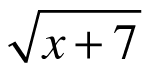+ 5 = x

2.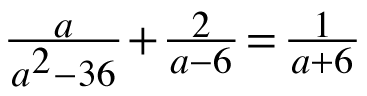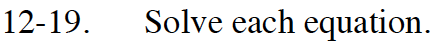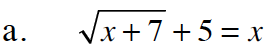Subtract 5 from both sides to isolate the radical.

$\sqrt{x+7}\ =\ x-5$

Square both sides to get rid of the radical sign.

$x+7\ =\ x^2-10x+25$

Set the equation equal to 0 and usethe Quadratic Formula to solve for x.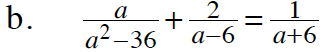What are the factors of a2 − 36? Multiply both sides of the equation by a common denominator to remove the fractions.

a = −9